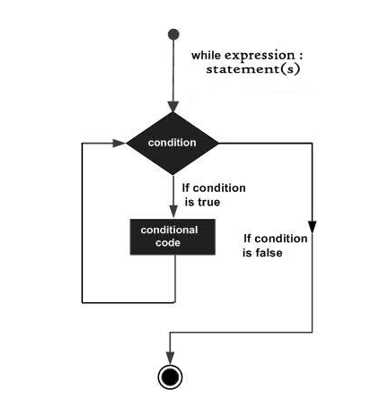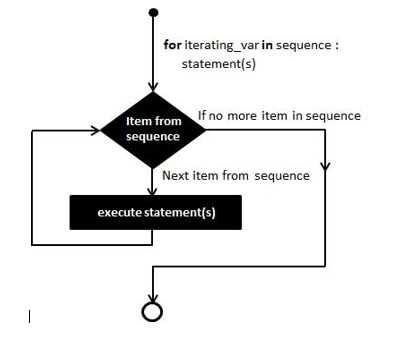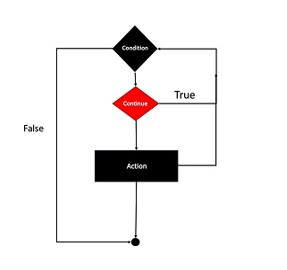# Loop in PHP

Loop is used when we want to execute a same code for several times.

In PHP we have 4 types of loop:

##### While loop:

Loops through a block of code till the condition is true. The while loop execute the code till the test expression is true. For example:When the condition is true code will execute and when it becomes false it will terminate.

```<?php \$a = 1;while(\$a <= 5) {  echo 'The number is: \$a<br>';  \$a++;
}
?> ```

The number is: 1

The number is: 2

The number is: 3

The number is: 4

The number is: 5

##### For loop

For loop executed for a specified number of times.The following example will iterate for 5 times

```<?phpfor (\$a = 1; \$a <= 5; \$a++) {//Code will execute 5 times    echo 'Hello World!! </br>';
}
?>
```

Hello World!!

Hello World!!

Hello World!!

Hello World!!

Hello World!!

##### The PHP do while loop

In do while code will execute atleast once,it will then check the condition and repeat the loop till the specified condition is true.

```<?php\$a = 1;
do {
echo 'Hello World!! <br>';
\$a++;} while (\$a <= 5);
?>```

Output:

Hello World!!

Hello World!!

Hello World!!

Hello World!!

Hello World!!

##### Foreach loop:

Foreach works only on array and for each pass the value of the current  array is assigned to \$text as shown in example:

```<?php\$day = array('Mon', 'Tue', 'Wed', 'Thu','Fri','Sat','Sun');
foreach (\$day as \$text=>\$value) {
echo '\$text:\$value <br>';}

?>```
Output:// By default From 0 to 6 the key is assigned to values.
0:Mon
1:Tue
2:Wed
3:Thu
4:Fri
5:Sat
6:Sun

The break Statement:

The break statement is used to terminate the statement prematurely.These type of statement are situated inside the statement.It gives you full control and whenever you want to come out from the statement you can by using break statement.

```<?php

\$text = array( 1, 2, 3, 4, 5);
foreach( \$text as \$value ) {            if( \$value == 3 )break;            echo 'Value is \$value <br />';
}
?>
```

Output:

Value is 1

Value is 2 //After \$value=3the loop will terminate.

##### The Countinue statement

Like the break statement the countinue is also written inside the loop.Both break and countinue are same except  countinue does not terminate the loop.```<?php
\$text = array( 1, 2, 3, 4, 5);          foreach( \$text as \$value ) {            if( \$value == 3 )continue;            echo 'Value is \$value <br />';
}
?>
```
Output://It will remove \$value = 3 and won’t terminate the loop unlike break statement
Value is 1
Value is 2
Value is 4
Value is 5
Last updated:9/7/2019 12:16:21 AM#### Samuel Fernandes